# Texas Go Math Grade 8 Lesson 2.2 Answer Key Scientific Notation with Negative Powers of 10

Refer to our Texas Go Math Grade 8 Answer Key Pdf to score good marks in the exams. Test yourself by practicing the problems from Texas Go Math Grade 8 Lesson 2.2 Answer Key Scientific Notation with Negative Powers of 10.

## Texas Go Math Grade 8 Lesson 2.2 Answer Key Scientific Notation with Negative Powers of 10

Essential Question
How can you use scientific notation to express very small quantities?

You can use what you know about writing very large numbers in scientific notation to write very small numbers in scientific notation.

A typical human hair has a diameter of 0.000025 meter. Write this number in scientific notation.

A. Notice how the decimal point moves in the list below. Complete the list.B. Move the decimal point in 0.000025 to the right as many places as necessary to find a number that is greater than or equal to 1 and less than 10. What number did you find? ____
D. Combine your answers to B and c to represent 0.000025 in scientific notation. ____

Reflect

Question 1.
When you move the decimal point, how can you know whether you are increasing or decreasing the number?
When the decimal point moves to the right, the number is increasing and if it moves to the left, the is decreasing.

Question 2.
Explain how the two steps of moving the decimal and multiplying by a power of 10 leave the value of the original number unchanged.
The power of 10 represents the number of times the decimal is moved, hence multiplying will leave the value of the original number unchanged.

Reflect

Question 3.
Critical Thinking When you write a number that is less than 1 in scientific notation, how does the power of 10 differ from when you write a number greater than 1 in scientific notation?
When we write a number that is less than 1 in scientific notation, the power of 10 is negative, whereas when we write a number that is greater than 1 in scientific notation, the power of 10 is positive

Write each number in scientific notation.

Question 4.
0.0000829
0.0000829 Given
8.29 Place the decimal point
5 Count the number of places the decimal point is moved.
8.29 × 10-5 Multiply 8.29 times a power of 10.
Since 0.0000829 is less than 1, the decimal point moves to the right and the exponent on 10 is negative.
8.29 × 10-5

Question 5.
0.000000302
0.000000302 Given
3.02 Place the decimal point
7 Count the number of places the decimal point is moved.
3.02 × 10-7 Multiply 3.02 times a power of 10. Since the original number is less than 1, the decimal point moves to the right and the exponent on 10 is negative.
3.02 × 10-7

Question 6.
A typical red blood cell in human blood has a diameter of approximately 0.000007 meter. Write this diameter in scientific notation. ________________________
0.000007 Given
7.0 Place the decimal point
6 Count the number of places the decimal point is moved.
7.0 × 10-6 Multiply 7.0 times a power of 10. Since the original number is less than 1, the decimal point moves to the right and the exponent on 10 is negative.
7.0 × 10-6

Reflect

Question 7.
Justify Reasoning Explain whether 0.9 × 10-5 is written in scientific notation. If not, write the number correctly in scientific notation.
In order for a number to be written in scientific notation they must be of the format:
c × 10n (1)
where c is a decimal number greater or equal to 1 and less than 10 and n is an integer
Since in the given number, 0.9 < 1 this number is not written in scientific notation.
A correct way to write that number in scientific notation would be to move the decimal point one place to the
right because of that the exponent would also decrease by one:
9× 10-6 (2)

Question 8.
Which number is larger, 2 × 10-3 or 3 × 10-2? Explain.
2 × 10-3 Given
and
3 × 10-2
3 × 10-2 is larger when comparing exponents, -2 > -3

Write each number in standard notation.

Question 9.
1.045 × 10-6
1.045 × 10-6 Given
6 Use the exponent of the power of 10 to see how many places to move the decimal point.
places
0.000001045 Place the decimal point Since you are going to write a number less than 1.045, move the decimal to the left. Add placeholder zeros if necessary.
The number
1.045 × 10-6 in standard notation is 0.000001045

Question 10.
9.9 × 10-5
9.9 × 10-5 Given
5 Use the exponent of the power of 10 to see how many places to move the decimal point.
places
0.000099 Place the decimal point Since you are going to write a number less than 9.9, move the decimal to the left. Add placeholder zeros if necessary.
The number
9.9 × 10-5 in standard notation is 0.000099

Question 11.
Jeremy measured the length of an ant as 1 × 10-2 meter. Write this length in standard notation.
1 × 10-2 Given
2 Use the exponent of the power of 10 to see how many places to move the decimal point.
places
0.01 Place the decimal point Since you are going to write a number less than 1, move the decimal to the left. Add placeholder zeros if necessary.
The number
1 × 10-2 in standard notation is 0.01 meter
0.01

Write each number in scientific notation. (Explore Activity and Example 1)

Question 1.
0.000487
Hint: Move the decimal right 4 places.
0.000487 Given
4.87 Place the decimal point
4 Count the number of places the decimal point is moved.
4.87 × 10-4 Multiply 4.87 times a power of 10.
Since 0.000487 is less than 1, the decimal point moves to the right and the exponent on 10 is negative.
4.87 × 10-4

Question 2.
0.000028
Hint: Move the decimal right 5 places.
Move the decimal point as many places as necessary to find a number that is greater that or equal to 1 and less
than 10:
2.8 Place the decimal point (1)
5 places Count the number of places you moved the decimal point (2)
2.8 × 10-5 Multiply the number from step (1) with 10-5 (3)
Note: in step (3) you multiplied 2.8 with 10-5 because you moved the decimal point 5 places to the right
2.8 × 10-5

Question 3.
0.000059
0.000059 Given
5.9 Place the decimal point
5 Count the number of places the decimal point is moved.
5.9 × 10-5 Multiply 5.9 times a power of 10. Since 0.000059 is less than 1, the decimal point moves to the right and the exponent on 10 is negative
5.9 × 10-5

Question 4.
0.0417
0.0417 Given
4.17 Place the decimal point
2 Count the number of places the decimal point is moved.
4.17 × 10-2 Multiply 4.17 times a power of 10. Since 0.0417 is less than 1, the decimal point moves to the right and the exponent on 10 is negative
4.17 × 10-2

Question 5.
Picoplankton can be as small as 0.00002 centimeter.
0.00002 Given
2.0 Place the decimal point
5 Count the number of places the decimal point is moved.
2 × 10-5 Multiply 2 times a power of 10. Since 0.00002 is less than 1, the decimal point moves to the right and the exponent on 10 is negative
2 × 10-5 centimeter

Question 6.
The average mass of a grain of sand on a beach is about 0.000015 gram.
0.000015 gram Given
1.5 Place the decimal point
5 Count the number of places the decimal point is moved.
1.5 × 10-5 Multiply 1.5 times a power of 10. Since 0.000015 is less than 1, the decimal point moves to the right and the exponent on 10 is negative
1.5 × 10-5 gram

Write each number in standard notation. (Example 2)

Question 7.
2 × 10-5
Hint: Move the decimal left 5 places.
2 × 10-5 Given
5 Use the exponent of the power of 10 to see how many places to move the decimal point.
places
0.00002 Place the decimal point. Since you are going to write a number less than 2, move the decimal to the left. Add placeholder zeros if necessary.
The number
2 × 10-5 in standard notation is 0.00002

Question 8.
3.582 × 10-6
Hint: Move the decimal left 6 places.
3.582 × 10-6 Given
6 Use the exponent of the power of 10 to see how many places to move the decimal point.
places
0.000003582 Place the decimal point. Since you are going to write a number less than 3.582, move the decimal to the left. Add placeholder zeros if necessary.
The number
3.582 × 10-6 in standard notation is 0.000003582

Question 9.
8.3 × 10-4
8.3 × 10-4 Given
4 Use the exponent of the power of 10 to see how many places to move the decimal point.
places
0.00083 Place the decimal point. Since you are going to write a number less than 8.3, move the decimal to the left. Add placeholder zeros if necessary.
The number
8.3 × 10-4 in standard notation is 0.00083

Question 10.
2.97 × 10-2
2.97 × 10-2 Given
2 Use the exponent of the power of 10 to see how many places to move the decimal point.
places
0.0279 Place the decimal point. Since you are going to write a number less than 2.97, move the decimal to the left. Add placeholder zeros if necessary.
The number
2.7 × 10-2 in standard notation is 0.0297

Question 11.
9.06 × 10-5
9.06 × 10-5 Given
5 Use the exponent of the power of 10 to see how many places to move the decimal point.
places
0.0000906 Place the decimal point. Since you are going to write a number less than 9.06, move the decimal to the left. Add placeholder zeros if necessary.
The number
9.06 × 10-5 in standard notation is 0.0000906

Question 12.
4 × 10-5
(1) First, use the exponent of the power of 10 to see how many places to move the decimal point. Since we have 10-5 we have to move the decimal point 5 places.
(2) Place the decimal point, since you are going to write a number less than 4, move the decimal point to the left Add placeholder zeros if necessary:
4 × 10-5 = 0.00004.
0.00004

Question 13.
The average length of a dust mite is approximately 0.0001 meter. Write this number in scientific notation. (Example 1)
Move the decimal point as many places as necessary to find a number that is greater than or equal to 1 and less
than 10:
1 Place the decimal point (1)
4 places Count the number of places you moved the decimal point (2)
1 × 10-4 Multiply the number from step (1) with 10-4 (3)
Note: in step (3) you multiplied 1 with 10-4 because you moved the decimal point 5 places to the right because 0.0001 is smaller than 1.
1 × 10-4 meter.

Question 14.
The mass of a proton is about 1.7 × 10-24 gram. Write this number in standard notation. (Example 2)
1.7 × 10-24 gram Given
24 Use the exponent of the power of 10 to see how many places to move the decimal point
places

0.0000000000000000000000017 Place the decimal point Since you are going to write a number less than 1.7, move the decimal to the left Add placeholder zeros if necessary.
The number
1.7 × 10-24 in
standard notation is
0.0000000000000000000000017 gram

Essential Question Check-In

Question 15.
Describe how to write 0.0000672 in scientific notation.
(1) Place the decimal point such that the new number is larger or equal to 1 but less than 10.
0.0000672 ⇒ 6.72
(2) Count the number of places you moved the decimal. point: 5 places.
(3) Multiply 6.72 by 10-5 (because you moved the decimal 5 places to the right the exponent is negative)
6.72 × 10-5

Use the table for problems 16-21. Write the diameter of the fibers in scientific notation.Question 16.
Alpaca
__________
0.00277 Fiber diameter of Alpaca
2.77 Place the decimal point
3 Count me number 0f places the decimal point is moved.
2.77 × 10-3 Multiply 2.77 times a power of 10. Since 0.00277 is less than 1, the decimal point moves to the right and the exponent on 10 is negative.
2.77 × 10-3

Question 17.
Angora rabbit
_________
0.0013 Fiber diameter of Angora rabbit
1.3 Place the decimal point
3 Count me number 0f places the decimal point is moved.
1.3 × 10-3 Multiply 1.3 times a power of 10. Since 0.0013 is less than 1, the decimal point moves to the right and the exponent on 10 is negative.
1.3 × 10-3

Question 18.
Llama
_____
0.0035 Fiber diameter of Llama
3.5 Place the decimal point
3 Count me number 0f places the decimal point is moved.
3.5 × 10-3 Multiply 3.5 times a power of 10. Since 0.0035 is less than 1, the decimal point moves to the right and the exponent on 10 is negative.
3.5 × 10-3

Question 19.
Angora goat
______
0.0045 Fiber diameter of Angora goat
4.5 Place the decimal point
3 Count me number 0f places the decimal point is moved.
4.5 × 10-3 Multiply 4.5 times a power of 10. Since 0.0045 is less than 1, the decimal point moves to the right and the exponent on 10 is negative.
4.5 × 10-3

Question 20.
Orb web spider
0.015 Fiber diameter of Orb web spider
1.5 Place the decimal point
2 Count me number 0f places the decimal point is moved.
1.5 × 10-2 Multiply 1.5 times a power of 10. Since 0.0045 is less than 1, the decimal point moves to the right and the exponent on 10 is negative.
1.5 × 10-2

Question 21.
Vicuna
0.0008 Fiber diameter of Vicuna
8.0 Place the decimal point
4 Count me number 0f places the decimal point is moved.
8.0 × 10-4 Multiply 8.0 times a power of 10. Since 0.0008 is less than 1, the decimal point moves to the right and the exponent on 10 is negative.
8.0 × 10-4

Question 22.
Make a Conjecture Which measurement would be least likely to be written in scientific notation: the thickness of a dog hair, the radius of a period on this page, the ounces in a cup of milk? Explain your reasoning.
Both the thickness of a dog hair and the radius of a period on a page are very small, numbers (Lengths), something that we tend to write in scientific notation (because it is easier to work with when written in scientific notation). On the other hand, it is likely that the number of ounces in a cup of milk is a number that we can easily write and work with, so we don’t need to write it in scientific notation, so it is reasonable to suspect that it is the least likely to be written in scientific notation.
The number of ounces in a cup of milk

Question 23.
Multiple Representations Convert the length 7 centimeters to meters. Compare the numerical values when both numbers are written in scientific notation.
There are 100 centimeters in 1 meter, or 1 centimeter = 0.01 meters
Because of this 7 centimeters = 0.07 meters
Write 0.07 in scientific notation by moving the decimal point two places to the right:
0.07 = 7 × 10-2 meter
Since 7 centimeters is a number already greater or equal to 1 and less than 10 to get a scientific notation we just
multiply it with 100 = 1
7 = 7 × 100 centimeters
Finally we can see that when in scientific notation the first factor is the same but the exponents of the second factor differ by 2.

In scientific notation the first factor is the same but the exponents of the second factor differ by 2.

Question 24.
Draw Conclusions A graphing calculator displays 1.89 × 10-12 as 1.89E12. How do you think it would display 1.89 × 10-12? What does the E stand for?
We are told that 1.89. 1012 will be displayed as 1.89e12 in a scientific calculator.
We can conclude that here e stands for exponent of 10 and 1.89 . 10-12 will be displayed as 1.89e -12

Question 25.
Communicate Mathematical Ideas When a number is written in scientific notation, how can you tell right away whether or not it is greater than or equal to 1 ?
In a scientific notation, if the exponent of 10 is negative, the number is smaller than 1. Otherwise, it is greater or equal to 1.

Question 26.
The volume of a drop of a certain liquid is 0.000047 liter. Write the volume of the drop of liquid in scientific notation.
0.000047 liter Given
4.7 Place the decimal point
5 Count the number of places the decimal point is moved.
4.7 × 10-5 Multiply 4.7 times a power of 10. Since 0.000047 is less than 1, the decimal point moves to the right and the exponent on 10 is negative.
4.7 × 10-5

Question 27.
Justify Reasoning If you were asked to express the weight in ounces of a ladybug in scientific notation, would the exponent of the 10 be positive or negative? Justify your response.
Since the weight of a ladybug in ounces ¡s a number smaller than 1 we would need to move the decimal point to the right. Therefore the exponent of the 10 would be a negative number.

Negative, since the weight of a ladybug is smaller than 1.

Physical Science The table shows the length of the radii of several very small or very large items. Complete the table.

Question 28.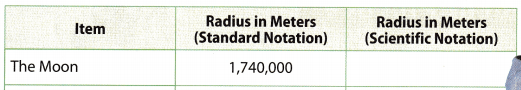(1) Move the decimal point to the left and remove extra zeros:
1,740,000 ⇒ 1.74
(2) Count the number of places you moved the decimal. point: 6 places
(3) Multiply 1.74 by 106 (because we moved the decimal point 6 places to the left 1.74 × 106
1.74 × 106 meters

Question 29.Question 30.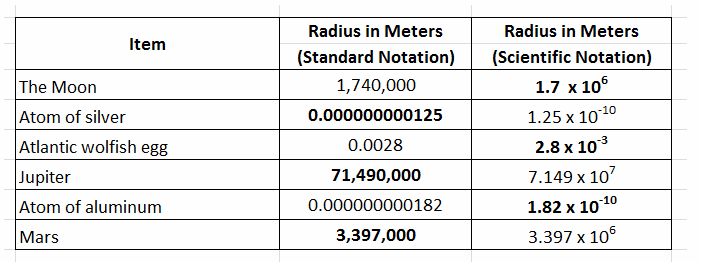Question 31.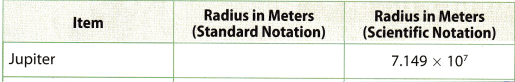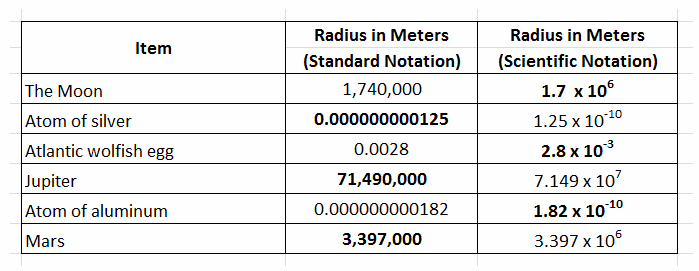Question 32.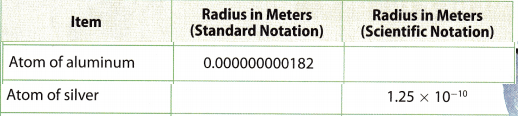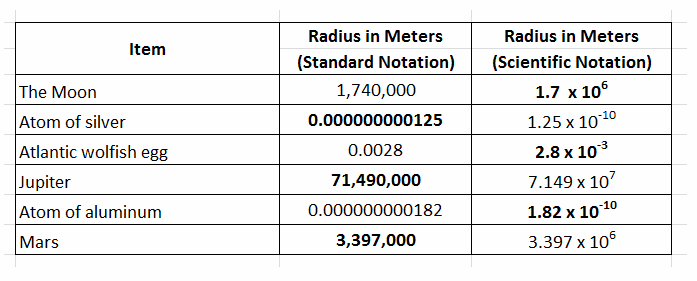Question 33.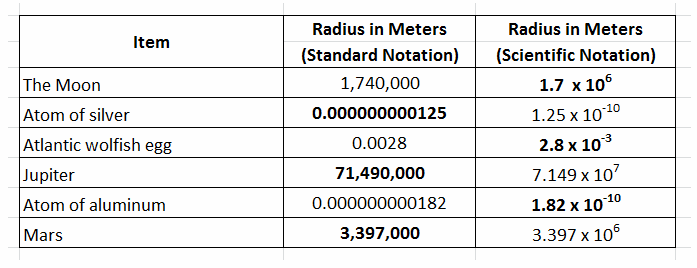Question 34.
List the items in the table in order from the smallest to largest.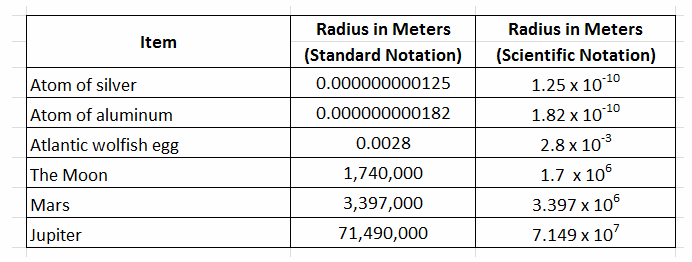Compare the powers of 10 and when they are same, compare the first factor. Based on this rule, the items are arranged from smallest to largest.

Texas Go Math Grade 8 Lesson 2.2 H.O.T. Focus On Higher Order Thinking Answer Key

Question 35.
Analyze Relationships Write the following diameters from least to greatest. 1.5 × 10-2m 1.2 × 102m 5.85 × 10-3 m 2.3 × 10-2 m 9.6 × 10-1 m
Compare the powers of 10 (smaller the exponent the smaller the number), if some are the same then compare the
first factors:
5.85 × 10-3 < 1.5 × 10-2 < 2.3 × 10-2 < 9.6 × 10-1 < 1.2 × 102

5.85 × 10-3, 1.5 × 10-2, 2.3 × 10-2, 9.6 × 10-1, 1.2 × 102

Question 36.
Critique Reasoning Jerod’s friend Al had the following homework problem:
Express 5.6 × 10-7 in standard form.
Al wrote 56,000,000. How can Jerod explain Al’s error and how to correct it?
5.6 × 10-7 Given
Error is that the decimal is moved in the moved in right direction and not left direction.
Since the exponent of 10 is -7, the decimal should be moved to left.

7 Use the exponent of the power of 10 to see how many places to move the decimal point
places
0.00000056 Place the decimal point Since you are going to write a number less than 5.6, move the decimal to the left. Add placeholder zeros if necessary.

Question 37.
Make a Conjecture Two numbers are written in scientific notation. The number with a positive exponent is divided by the number with a negative exponent. Describe the result. Explain your answer.
$$\frac{a^{m}}{a^{n}}$$ = am-n
$$\frac{a \times 10^{m}}{b \times 10^{-n}}$$ = $$\frac{a}{b}$$ × 10m+n